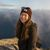# Attribute rule to create polygon feature from line features.

213
1
03-23-2023 04:13 PM
Labels (3)New Contributor

Hello,

I am hoping someone might be able to help me with writing an attribute rule that will generate polygons in a feature class triggered by the creation of lines that form closed areas in another feature class.

I imagine this rule would work by looking at whether the line created closes a shape (based on some topology) and if 'yes' then a polygon is created if 'no' then a polygon is not created.

This rule would be a huge help for those of us creating maps that would like to see what our maps are looking like in real time rather than having to run the features to polygons tool over and over.

There would need to be some other attributes for the polygons, but I thought I'd start with generating a polygon first.

Tags (3)byMVP Regular Contributor

You could do something like this:

``````// Calculation Attribute Rule on the Line fc
// field: leave empy
// triggers: insert
// exclude from application evaluation

// get the vertices
var vertices = Geometry(\$feature).paths

// if first vertex isn't the same as last vertex, abort
var same = vertices.x == vertices[-1].x && vertices.y == vertices[-1].y
if(!same) { return }

// create the polygon
var sr = Geometry(\$feature).spatialReference
var poly_vertices = []
for(var v in vertices) {
var vertex = [vertices[v].x, vertices[v].y]
Push(poly_vertices, vertex)
}
var poly = Polygon({rings: [poly_vertices], spatialReference: sr})

// insert the polygon into the polygon fc
return {
edit: [{
className: "NameOfYourPolygonFC",